Courses

# Olympiad Test : Mirror Images - 2

## 20 Questions MCQ Test Science Olympiad for Class 5 | Olympiad Test : Mirror Images - 2

Description
This mock test of Olympiad Test : Mirror Images - 2 for Class 5 helps you for every Class 5 entrance exam. This contains 20 Multiple Choice Questions for Class 5 Olympiad Test : Mirror Images - 2 (mcq) to study with solutions a complete question bank. The solved questions answers in this Olympiad Test : Mirror Images - 2 quiz give you a good mix of easy questions and tough questions. Class 5 students definitely take this Olympiad Test : Mirror Images - 2 exercise for a better result in the exam. You can find other Olympiad Test : Mirror Images - 2 extra questions, long questions & short questions for Class 5 on EduRev as well by searching above.
QUESTION: 1

Solution:
QUESTION: 2

Solution:
QUESTION: 3

### Select the one Correct option which exactly matches with the mirror image of the word /number figure given in the question.Solution:
QUESTION: 4

Select the one Correct option which exactly matches with the mirror image of the word /number figure given in the question.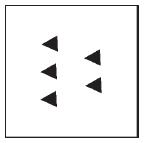Solution:
QUESTION: 5

Select the one Correct option which exactly matches with the mirror image of the word /number figure given in the question.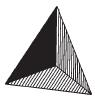Solution:
QUESTION: 6

Select the one Correct option which exactly matches with the mirror image of the word /number figure given in the question.
JAIDEEP

Solution:
QUESTION: 7

Select the one Correct option which exactly matches with the mirror image of the word /number figure given in the question.
SHARMA

Solution:
QUESTION: 8

Select the one Correct option which exactly matches with the mirror image of the word /number figure given in the question.
DBZ 29564

Solution:
QUESTION: 9

Select the one Correct option which exactly matches with the mirror image of the word /number figure given in the question.
V & S Publishers

Solution:
QUESTION: 10

​Select the one Correct option which exactly matches with the mirror image of the word /number figure given in the question.
Q. Looking into a mirror, the clock showed 2: 30 as the time. The actual time at that time was

Solution:
QUESTION: 11

​​Select the one Correct option which exactly matches with the mirror image of the word /number figure given in the question.
Q. Which set of letters look same in the mirror?

Solution:
QUESTION: 12

​​Select the one Correct option which exactly matches with the mirror image of the word /number figure given in the question.
Q. Which of the answer figure is exactly the mirror image of the problem figure?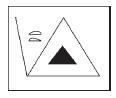Solution:
QUESTION: 13

​​Select the one Correct option which exactly matches with the mirror image of the word /number figure given in the question.
Q. If a mirror is placed on the line PQ, then which option is the right mirror image of given figure?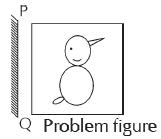Solution:
QUESTION: 14

Select the one Correct option which exactly matches with the mirror image of the word /number figure given in the question.
Q. If a mirror is placed on the line AB, then which of the answer figures is the right image of the given problem figure?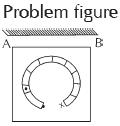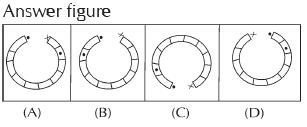Solution:
QUESTION: 15

​​​Select the one Correct option which exactly matches with the mirror image of the word /number figure given in the question.
Q. Which of the following set of letters will look same in the mirror

Solution:
QUESTION: 16

​​​Select the one Correct option which exactly matches with the mirror image of the word /number figure given in the question.
Q. How many capital letters of the English alphabet appear same when looked at in a mirror?

Solution:
QUESTION: 17

​​​​Select the one Correct option which exactly matches with the mirror image of the word /number figure given in the question.
Q. On looking into a mirror, it appears that it is quarter past three. What is the actual time?

Solution:
QUESTION: 18

​​​​Select the one Correct option which exactly matches with the mirror image of the word /number figure given in the question.
Q. What is the correct mirror image of following letter?
BIRTHDAY

Solution:
QUESTION: 19

Select the one Correct option which exactly matches with the mirror image of the word /number figure given in the question.
Q. What is the correct mirror image of the following number?
S2U5N9D4A6Y

Solution:
QUESTION: 20

Select the one Correct option which exactly matches with the mirror image of the word /number figure given in the question.
Q. Which one is the correct mirror image of the following figure?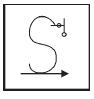Solution: